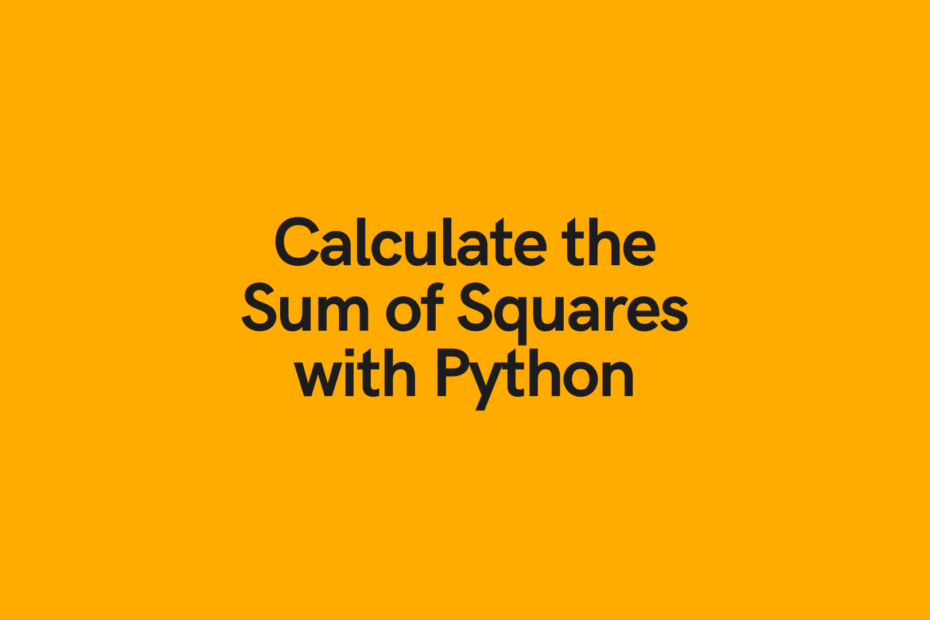# Python Sum of Squares: 3 Different WaysIn this post, you’ll learn different ways to calculate a Python sum of squares. You’ll learn different methods of calculating the sum of squares in order to find the most efficient method. You’ll also learn how to calculate the sum of squares between two different integers.

## What is the Sum of Squares?

The sum of squares refers to the sum of the squared numbers in a range of numbers. So, say you wanted to find the sum of squares of the numbers from 1 through N, this would be represented by:

``1² + 2² + 3² + 4².....+ N²``

The sum of squares represents a measure of variation and can be used to calculate the deviation from a mean.

## Python Sum of Squares with a For Loop

Now, the most intuitive way may be to calculate the Python sum of squares using a for loop. If you wanted a refresher on Python for-loops, check out my post here.

Say we want to calculate the sum of squares for the first 5 numbers, we can write:

``````sum_of_squares = 0

for num in range(6):
sum_of_squares += num ** 2

print(sum_of_squares)

# Returns: 55``````

What we’ve done here is created a variable `sum_of_squares` and assigned it the value of 0. We then loop over a range of numbers and add each numbers square to the variable `sum_of_squares`.

We can easily turn this into a function by writing:

``````def sum_of_squares_for_loop(end_number):
sum_of_squares = 0
for num in range(end_number + 1):
sum_of_squares += num ** 2
return sum_of_squares

print(sum_of_squares_for_loop(5))

# Returns: 55``````

## Python Sum of Squares with a List Comprehension

As with many for-loops, we can make them more Pythonic by refactoring them into a list comprehension. We can do this as well for calculating the sum of squares in Python.

Let’s see how this is done:

``````sum_of_squares = sum([num ** 2 for num in range(6)])
print(sum_of_squares)

# Returns: 55``````

If you want to learn more about Python list comprehensions, check out my tutorial here. I also have a detailed Youtube video you can check out here:

If you want to turn this method into a function, you can simply write:

``````def sum_of_squares_list_comprehension(end_number):
return sum([num ** 2 for num in range(end_number + 1)])

print(sum_of_squares_list_comprehension(5))
# Returns: 55``````

## Calculate Sum of Squares with a Formula

The above methods are fairly efficient and run at O(n), meaning that they scale based on the number of items passed into them. There is a much more efficient way of calculating the sum of squares, and that is to use the formula for calculating it.

This method is more efficient as it only needs to a run a single time, rather than looping over a list of items.

The formula for calculating the sum of squares of the first N numbers can be described as below:

``( n * ( n + 1 ) * ( 2n + 1 ) ) / 6``

Where n represents the number of digits to calculate.

The easiest way to calculate this is to turn this into a function:

``````def sum_of_squares_formula(end_number):
return ( end_number * ( end_number + 1 ) * ( 2 * end_number + 1 ) ) // 6

print(sum_of_squares_formula(5))

# Returns: 55``````

We specifically use `//` since we want to return the integer. If you want to learn more about the different types of division in Python, check out Python Division: Float and Integer Division.

## What is the most efficient method to calculate a Python Sum of Squares?

To find the most efficient method, we need to run this against some fairly large numbers.

Let’s create a Python decorator and see how long each of these methods takes. To learn more about decorators, check out the official documentation here.

``````import time

def time_it(func):
"""Print the runtime of a decorated function."""
def wrapper_time_it(*args, **kwargs):
start_time = time.perf_counter()
value = func(*args, **kwargs)
end_time = time.perf_counter()
run_time = end_time - start_time
print(f"Finished {func.__name__!r} in {run_time:.10f} seconds")
return value
return wrapper_time_it

@time_it
def sum_of_squares_for_loop(end_number):
sum_of_squares = 0
for num in range(end_number + 1):
sum_of_squares += num ** 2
return sum_of_squares

@time_it
def sum_of_squares_list_comprehension(end_number):
return sum([num ** 2 for num in range(end_number + 1)])

@time_it
def sum_of_squares_formula(end_number):
return ( end_number * ( end_number + 1 ) * ( 2 * end_number + 1 ) ) // 6

sum_of_squares_for_loop(100000000)
sum_of_squares_list_comprehension(100000000)
sum_of_squares_formula(100000000)

# Returns:
# Finished 'sum_of_squares_for_loop' in 25.6299132000 seconds
# Finished 'sum_of_squares_list_comprehension' in 50.8583192000 seconds
# Finished 'sum_of_squares_formula' in 0.0007121000 seconds
``````

While the time savings on small numbers are relatively marginal, once you get into larger calculations, the formula method is by far the fastest as it does not increase in runtime no matter how many values you throw at it!

## Sum of Squares between two Integers in Python

Finally, let’s find a way to calculate the sum of squares between two integers. The methods we’ve looked at so far start at 1, but this may not be optimal for what you want to do.

For this method, we can re-use either the for-loop or the list comprehension method and simply modify the range parameters.

Let’s see how we can calculate the sum of squares between, say, 11 and 14.

``````>>> print(sum([num ** 2 for num in range(11, 15)]))
630``````

To accomplish this by writing a function, we can write the following:

``````def sum_of_squares_list_comprehension(start_number, end_number):
return sum([num ** 2 for num in range(start_number, end_number + 1)])

print(sum_of_squares_list_comprehension(11, 14))``````

This also returns `630`.

## Conclusion

In this post, you learned how to calculate the Python sum of squares using different methods, including a for loop and a list comprehension. You also learned how to calculate the sum of squares using a formula to make it more efficient. Finally you learned which method is the most efficient, thereby saving you lots of time!

#### Nik Piepenbreier

Nik is the author of datagy.io and has over a decade of experience working with data analytics, data science, and Python. He specializes in teaching developers how to use Python for data science using hands-on tutorials.View Author posts

Tags: